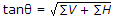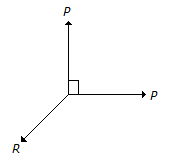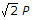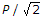# Mechanical Engineering - Engineering Mechanics

### Exercise :: Engineering Mechanics - Section 1

16.

Moment of inertia of a triangular section of base (b) and height (h) about an axis passing through its C.G. and parallel to the base, is

 A. bh3/4 B. bh3/8 C. bh3/12 D. bh3/36

Explanation:

No answer description available for this question. Let us discuss.

17.

If the masses of both the bodies, as shown in the below figure, are reduced to 50 percent, then tension in the string will beA. same B. half C. double

Explanation:

No answer description available for this question. Let us discuss.

18.

Which of the following is an equation of linear motion?(where, u and v = Initial and final velocity of the body, a = Acceleration of the body, and s = Displacement of the body in time t seconds.)

 A. v = u + a.t B. s = u.t + 1/2 a.t2 C. v2 = u2+2a.s D. all of these

Explanation:

No answer description available for this question. Let us discuss.

19.

If a number of forces are acting at a point, theirresultant will be inclined at an angle θ with the horizontal, such that

 A. tan θ = ∑H/∑V B. tan θ = ∑V/∑H C. tan θ = ∑Vx∑H D.Explanation:

No answer description available for this question. Let us discuss.

20.The above figure shows the two equal forces at right angles acting at a point. The value of force R acting along their bisector and in opposite direction is

 A. P/2 B. 2P C.D.